P66
3.1 浮点数运算结果只有前15个数字是确定正确的，整数运算能够输出完全正确的运算结果，使用整数可以获得精度更高的计算结果。（具体解释详见课本P64,65）
3.2 二进制：1111110010

``````#用format直接转化，format的用法在第一章有解释
print("{:b}".format(1010))    #二进制
print("{:o}".format(1010))    #八进制
print("{:x}".format(1010))    #十六进制
``````

3.3
-77.的科学计数法表示：-7.7e+1
4.3e-3的十进制表示：0.0043
3.4

``````print((2.3e+3-1.34e-3j).real)    #实数部分
print((2.3e+3-1.34e-3j).imag)    #虚数部分
``````

P69
3.5

``````print(30-3**2+8//3**2*10)   #21
print(3*4**2/8%5)   #1.0
print(2**2**3)      #256    #python中幂运算符**如果是多个的话，应该先算右边再算左边，也就是从右往左计算.2**3=8；2**8=256
print((2.5+1.25j)*4j/2)  #(-2.5+5j)
``````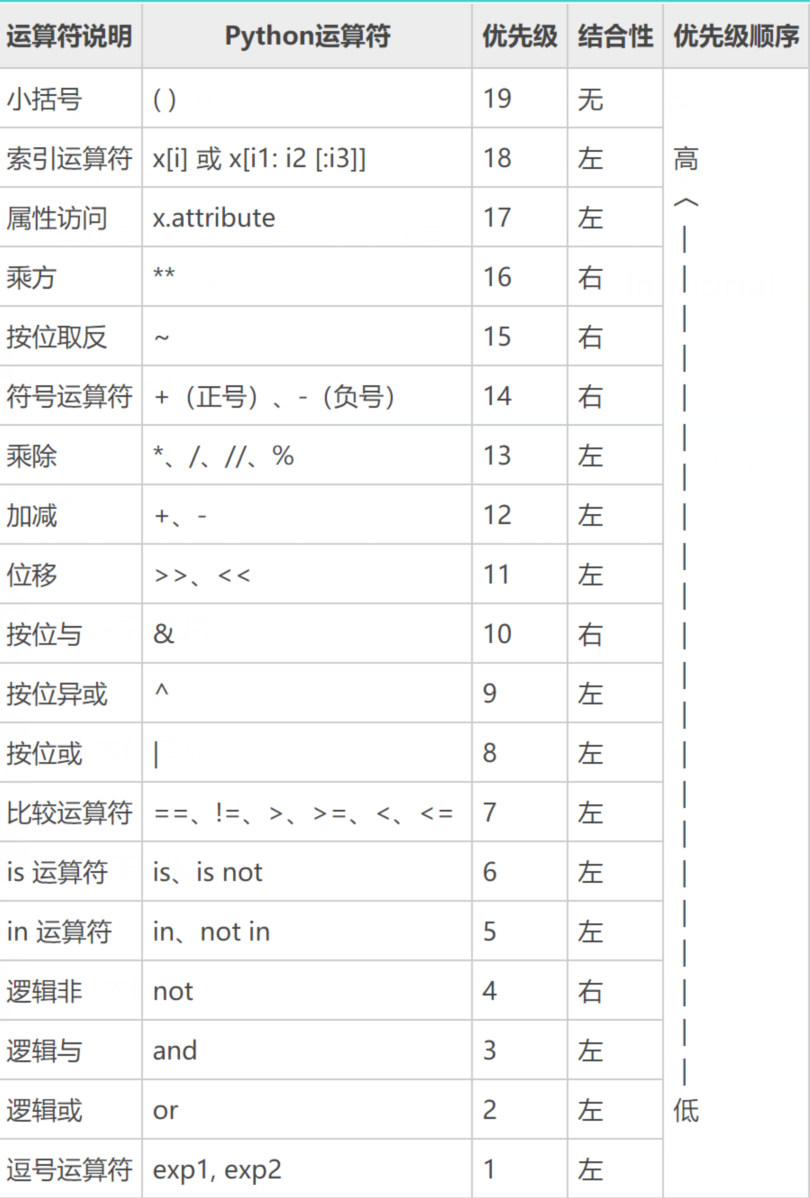3.6

``````print((2**4+7-3*4)/5)   #运算结果:2.2
print((1+3**2)*(16%7)/7)  #运算结果：2.857142857142857
``````

3.7

``````x = 1
x*=3+5**2  #x = x*(3+5**2)=1 * (3+25)=28
print(x)    #28
``````

P74
3.8

``````import math
print(math.sin(2*math.pi))  #-2.4492935982947064e-16     #pi常数π，sin正弦
print(math.floor(-2.5))     #-3     #floor(X)，向下取整，返回不大于X的最大整数
print(math.ceil(3.5+math.floor(-2.5)))  #1    #ceil(X)，向上取整，返回不小于X的最小整数
print(round(math.fabs(-2.5)))         #2      #fabs(X),X的绝对值    round(X)对X四舍五入
print(math.sqrt(math.pow(2,4)))      #4.0     #pow(x,y),x的y次幂,sqrt开平方
print(math.log(math.e))              #1.0     #e常数，自然对数：2.718281828459045   log(x),x的对数值
print(math.gcd(12,9))                #3       #gcd(x,y)x与y的最大公约数
print(math.fmod(36,5))               #1.0     #fmod(x,y):x%y
``````

3.9

``````import math
print(x)  #结果：0.8203047484373349
``````

3.10

``````import math
x = math.degrees(math.pi/7)   #degrees(x),x的弧度转角度
print(x)  #结果：25.714285714285715
``````

math常用的函数书上（P71-73）还有更多，但鉴于我以后可能懒得翻书，我决定贴上来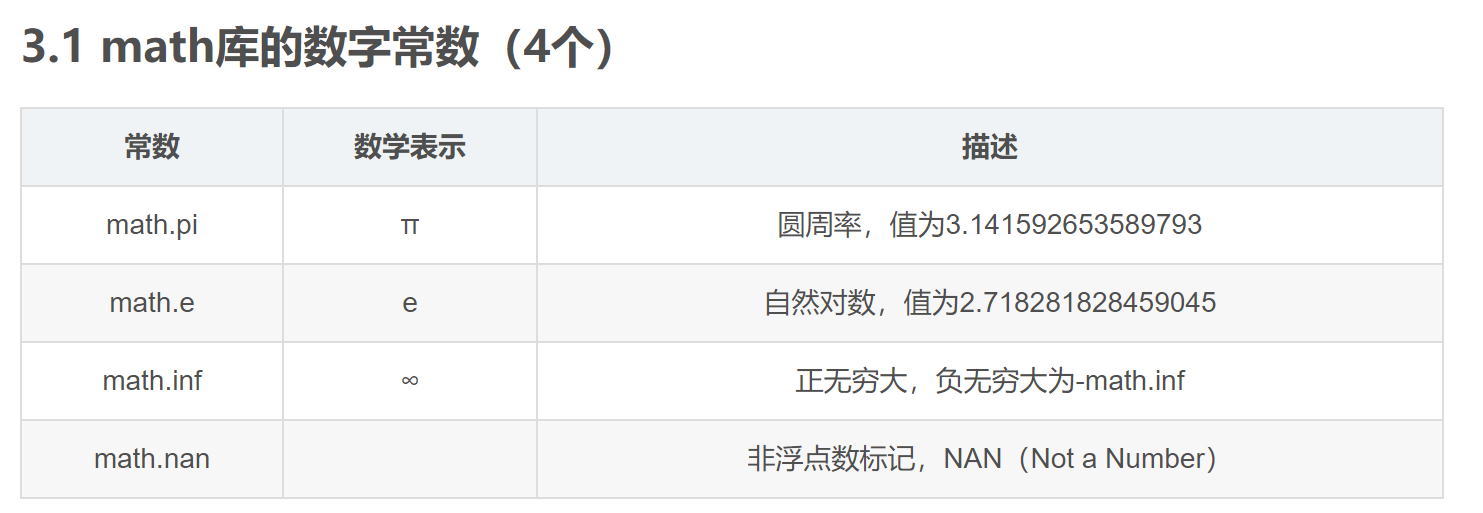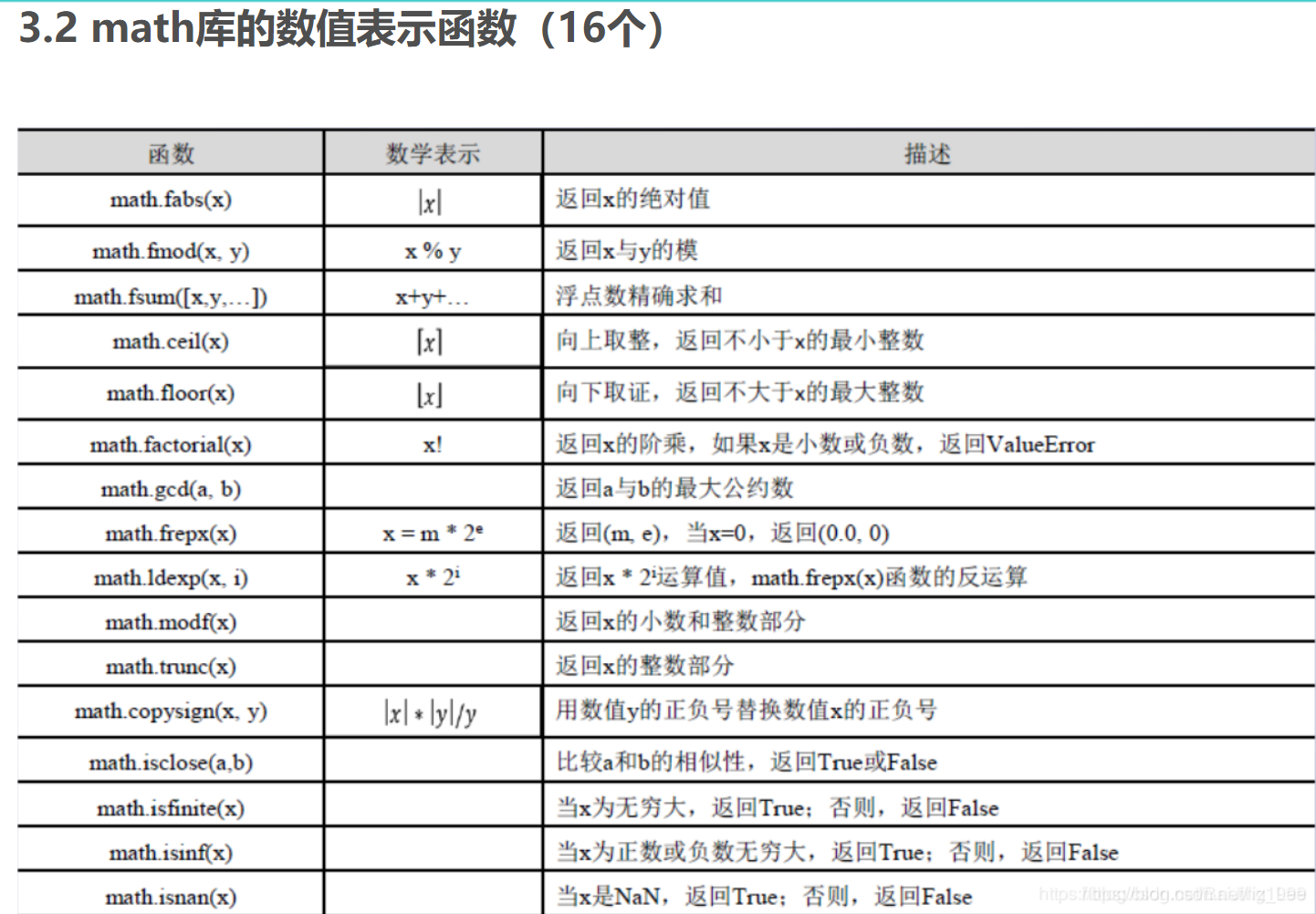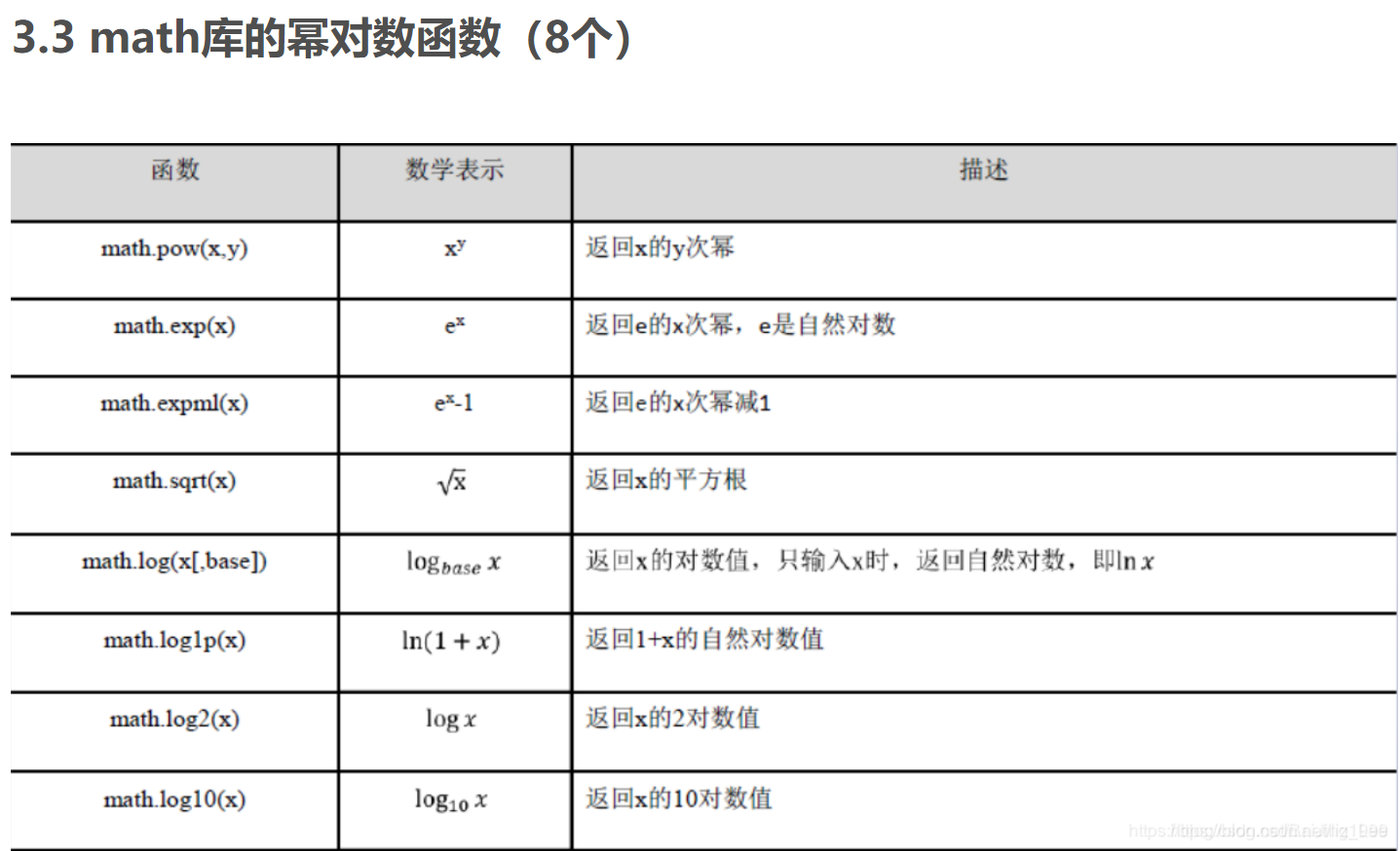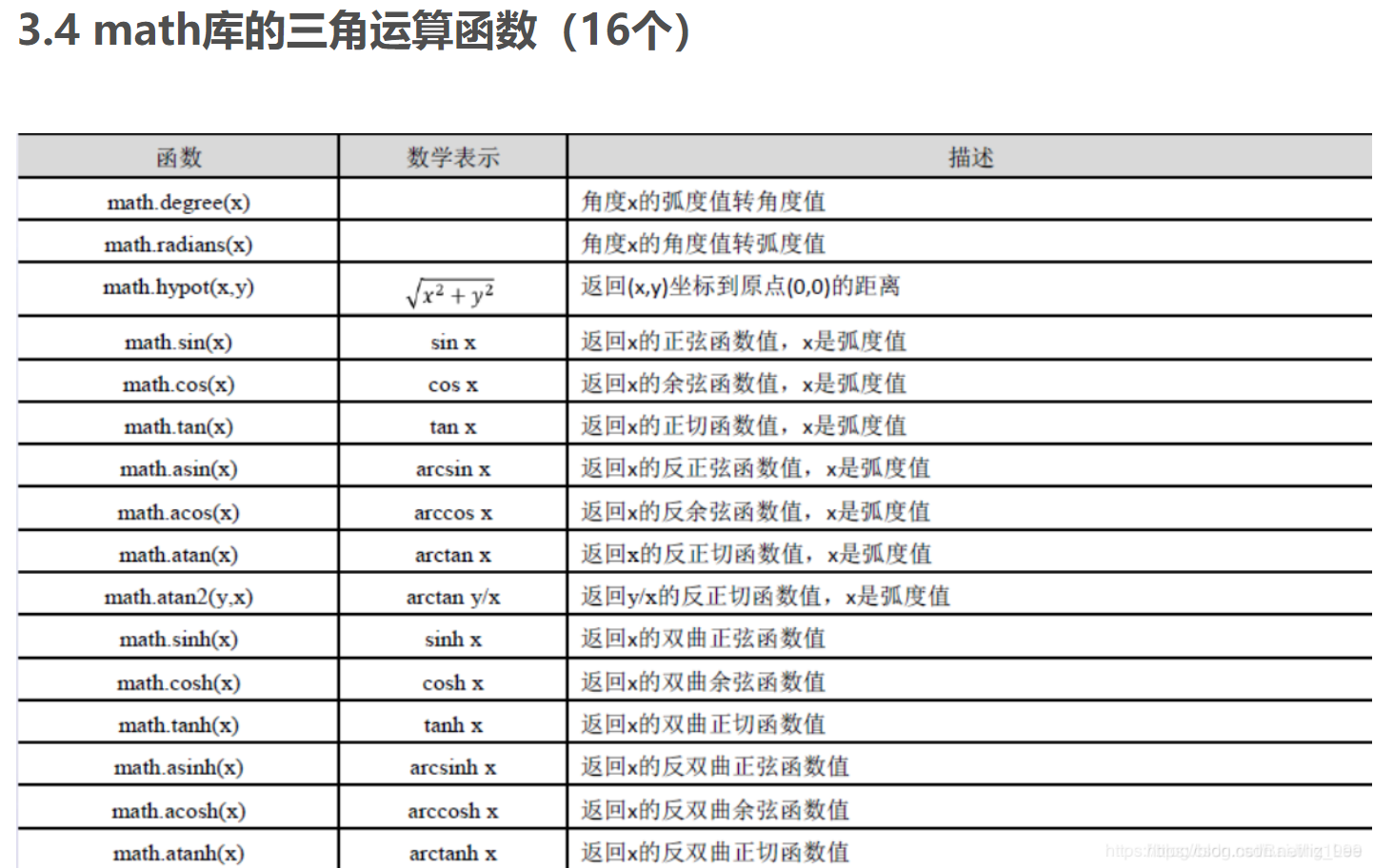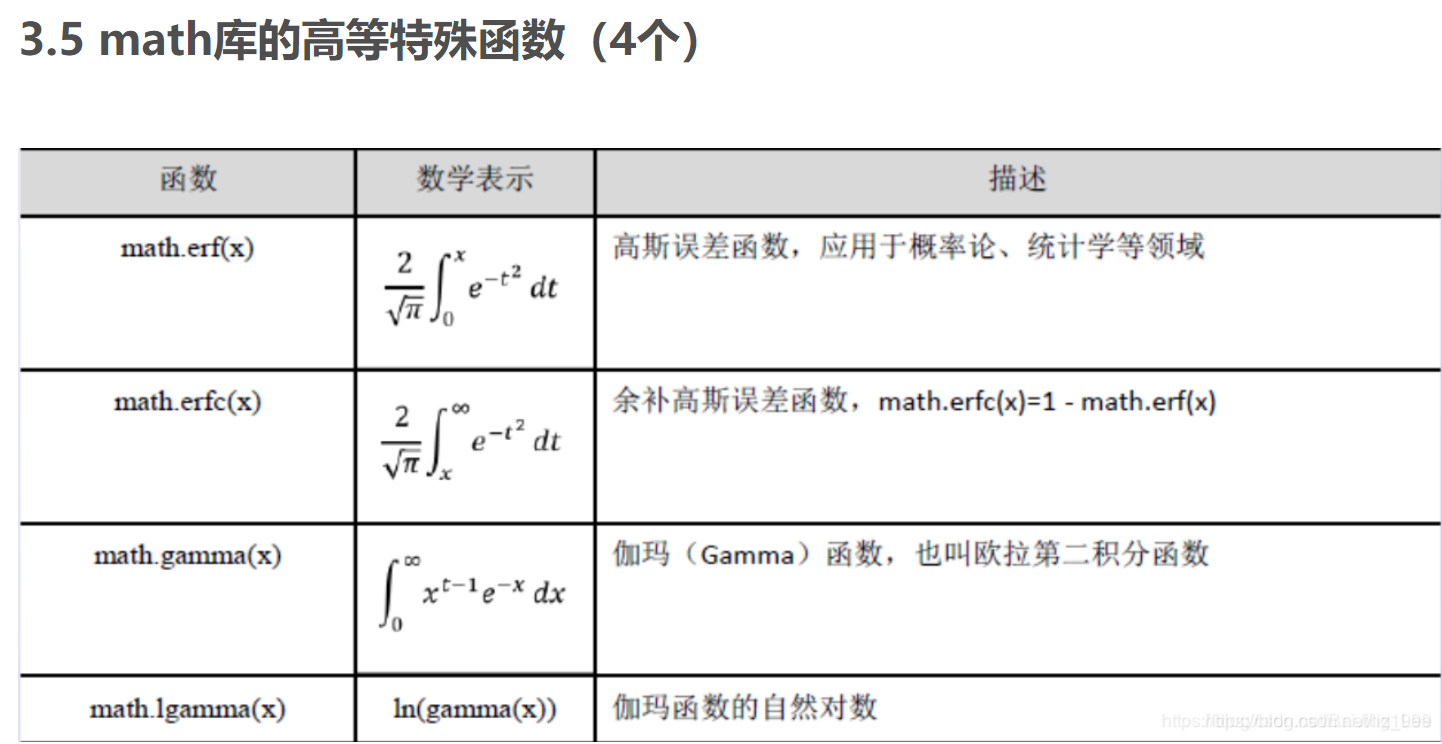3.11.

P77
3.12

``````dayup = 1.00     #初始水平值
dayfactor = 0.001   #每工作一天水平增加值
for j in range(10):
for i in range(1,365):
if i%7 in [1,2,3,4]:
dayup = dayup * (1+dayfactor)
else:
dayup = dayup
print("增加值为{:.3f}时年终值：{:.2f}".format(dayfactor,dayup))
dayfactor = dayfactor + 0.001
dayup = 1.00

``````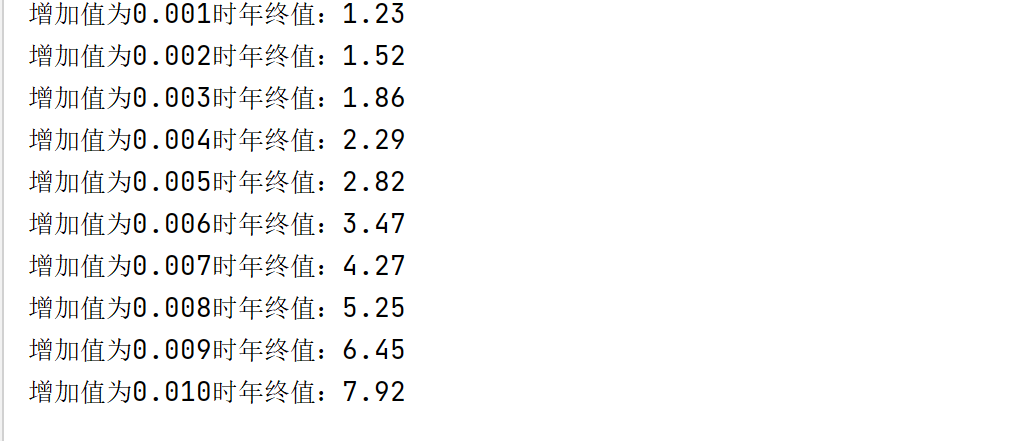3.13

``````dayup = 1.00     #初始水平值
dayfactor = 0.001   #每工作一天水平增加值
for j in range(10):
for i in range(1,365):
if i%7 in [1,2,3,4,5]:  #只增加了5。    #3.14一周工作六天，则再增加6
dayup = dayup * (1+dayfactor)
else:
dayup = dayup
print("增加值为{:.3f}时年终值：{:.2f}".format(dayfactor,dayup))
dayfactor = dayfactor + 0.001
dayup = 1.00
``````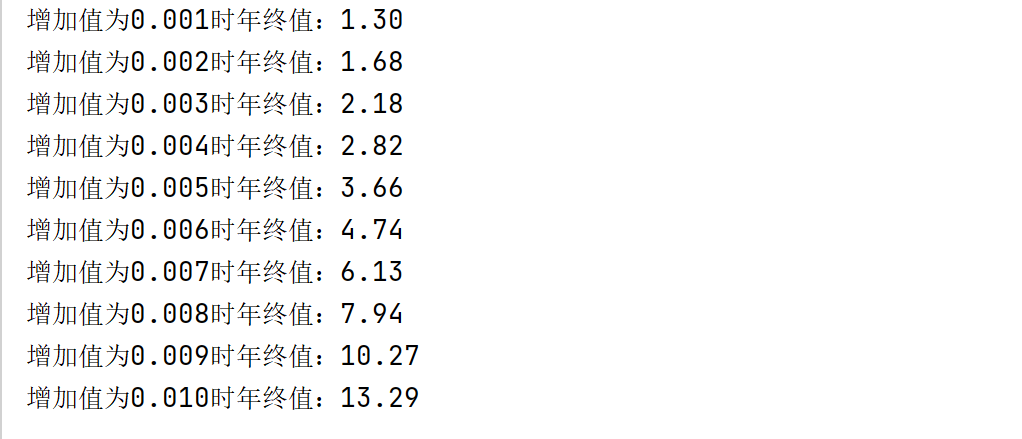3.14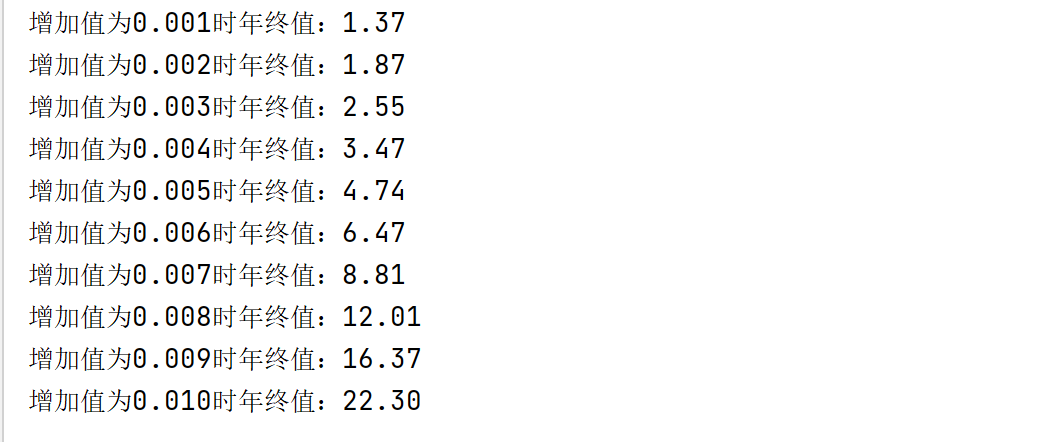3.15

``````dayup = 1.00
dayfactor = 0.001
for j in range(10):
for i in range(1,365):
if i%30 in [1,2,3,4,5,6,7,8,9,10]:
dayup = dayup * (1+dayfactor)
else:
dayup = dayup
print("增加值为{:.3f}时年终值：{:.2f}".format(dayfactor,dayup))
dayfactor = dayfactor + 0.001
dayup = 1.00
``````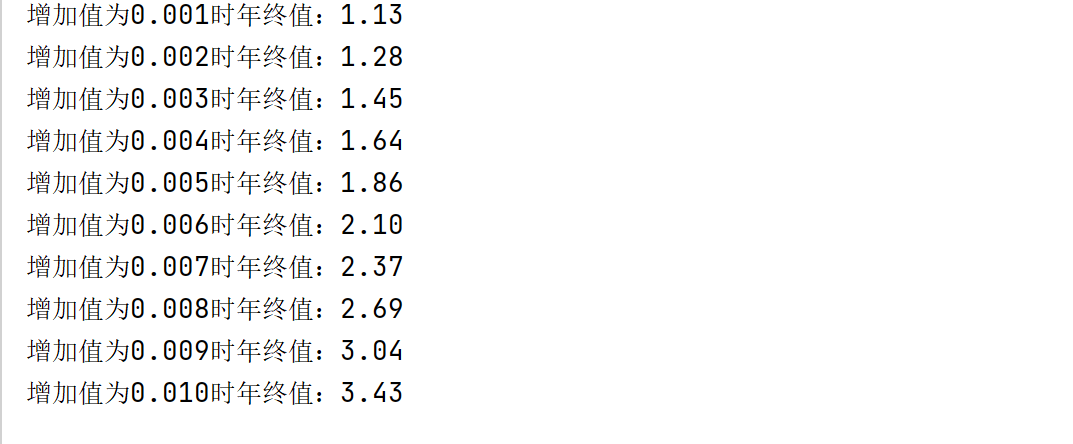P84
3.16

``````s = "hello"
t = "world"
s += t
print(s,'\n',s[-1],'\n',s[2:8],'\n',s[::3],'\n',s[-2::-1])
#运行结果：
# helloworld
#  d    [-1]倒数第一个
#  llowor   [2:8]  切片，从第二个到第七个，注意，从零开始
#  hlod   [::3]   从第一个开始，每隔三个
#  lrowolleh    [-2::-1] -2从倒数第二开始，-1倒序，这个表达式就是从倒数第二个开始倒序
``````

3.17

3.18

3.19

``````s = "Python String"
print(s.upper(),'\n',s.lower(),'\n',s.find('i'),'\n',s.replace('ing','gni'),'\n',s.split(' '))
#运算结果：
# PYTHON STRING       #upper()全部大写
#  python string      #lower()全部小写
#  10                 #find('X')，返回X的位置
#  Python Strgni      #replace(x,y),用y代替x
#['Python', 'String'] #split(x)转换成列表，由x分割
``````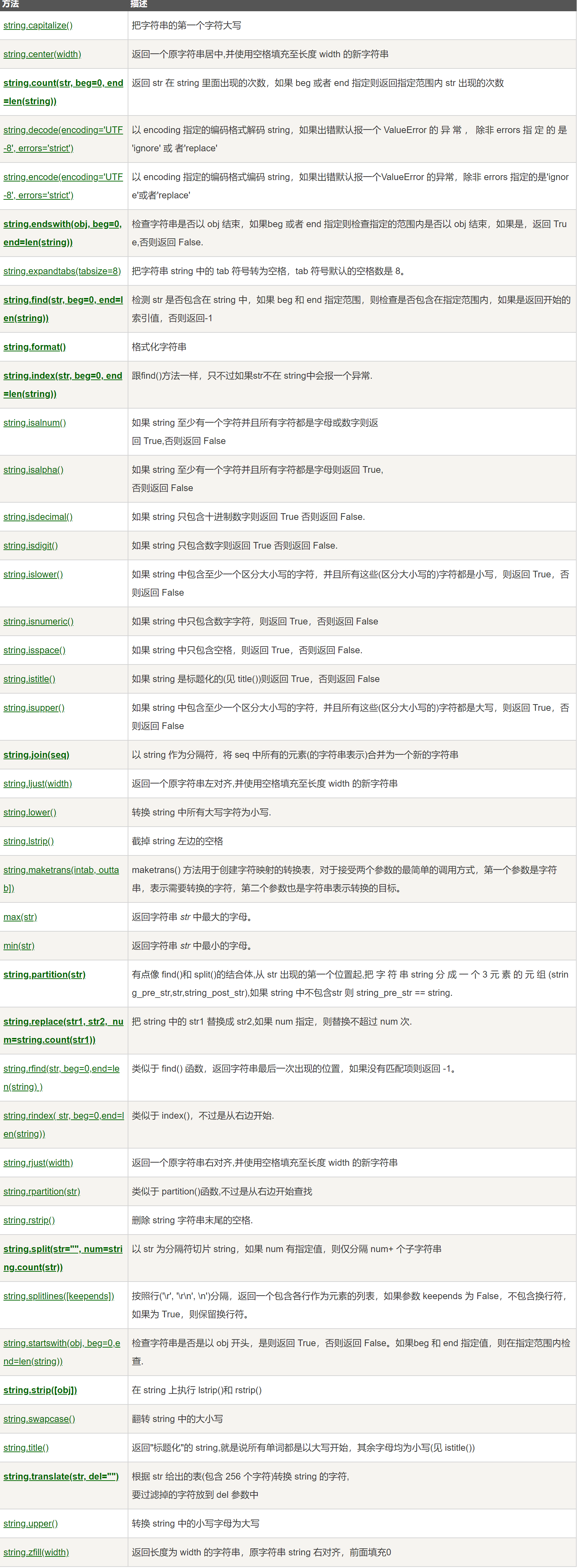3.20
CD
B选项:前三个元素都相等,但’abcd’有第三个元素,而’abc’没有.所以’abc’<‘abcd’.结果对
C选项:这个错了,既不是对,又不是错.
D选项:先比第0个元素,‘A’要比’a’小.所以’ABCD’应该小于’abcd’. 结果错误。
P88

3.21

``````print('{:>15s}:{:<8.2f}'.format('Length',23.87501))
#运行结果：
#         Length:23.88
``````

3.22

``````print('{:-^20b}'.format(389))    #输出二进制
print('{:-^20o}'.format(389))         #输出八进制
print('{:-^20d}'.format(389))           #输出十进制
print('{:-^20x}'.format(389))           #输出十六进制
print('{:-^20c}'.format(389))         #输出Unicode字符
#运行结果：
#-----110000101------
#--------605---------
#--------389---------
#--------185---------
#---------ƅ----------
``````

3.23

``````print("{:e}".format(0.002178))    #科学计数法
print("{:.4f}".format(0.002178))         #保留4位有效位的标准浮点形式
print("{:.2%}".format(0.002178))           #百分形式
#运行结果：
# 2.178000e-03
# 0.0022
# 0.22%
``````

P93
3.24

3.25
str.center()：居中字符串
3.26

``````print("\r你好Python")
print("\rPython语言程序设计基础")
print("你好\rPython")
print("Python语言程序设计基础\r")
# 你好Python
# Python语言程序设计基础
# Python
# Python语言程序设计基础
``````